Want to share your content on R-bloggers? click here if you have a blog, or here if you don't.

I just finished reading Paul Murrel’s book, “R graphics”.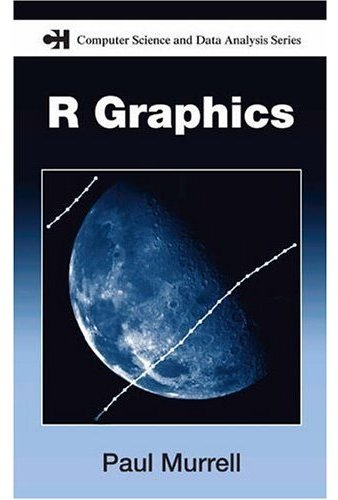There are two graphical systems in R: the old (“classical” —
in the “graphics” package) one, and the new (“trellis”, “lattice”,
“grid” — in the “lattice” and “grid” packages) one.

The first part of the book, devoted to the old system, tries to be
as complete as a reference book, but fails. For instance, the
discussion on how to arrange several plots on a single page sticks to
tabular layouts and fails to mention the more flexible “fig” graphical
argument (to be honest, it is listed with terser explanations than in
the manual page and also appears, deeply hidden in an example, in an
appendix).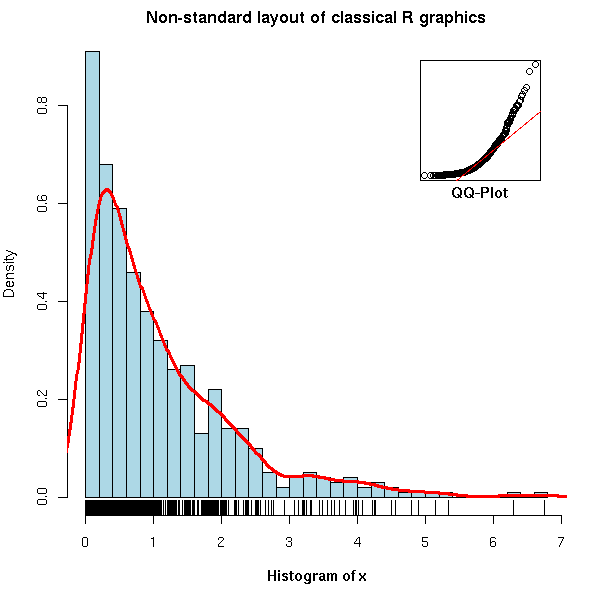```op <- par(mar = c(5,4,2,2))
N <- 500
x <- rexp(N, 1)
hist(x,
breaks = 30, probability = TRUE,
col = "light blue",
xlab = "",
main = "Non-standard layout of classical R graphics")
mtext(text = "Histogram of x",
side = 1, font = 2, line = 3)
lines(density(x),
col = "red", lwd = 3)
rug(x)
par(new = TRUE,
fig = c(.7, .9, .7, .9),
mar = c(0,0,0,0))
qqnorm(x,
axes = FALSE,
main = "", xlab = "", ylab = "")
box()
qqline(x,
col = "red")
par(xpd = NA)
mtext(text = "QQ-Plot",
line = .2, side = 1, font = 2)
par(op)```

On the other hand, the second and largest part, devoted to
grid graphics lives up to my expectations: it seems more
complete and does not duplicate information already
available on the web. You are probably already familiar with
some of the high-level lattice plots (xyplot(), histogram(),
bwplot()), but if you have already tried to understand how
they are implemented, or tried to write your own graphical
functions, you were probably confused by the differences
(and claimed lack thereof) between "lattice", "panel",
"grob" and "grid" -- the book clarifies all that.

The code of the examples in the book is available on the author's web site.

`http://www.stat.auckland.ac.nz/~paul/RGraphics/rgraphics.html`

You will find, for instance, dendrograms (check the rpart and maptree
packages),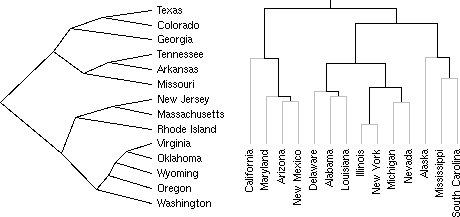table-like plots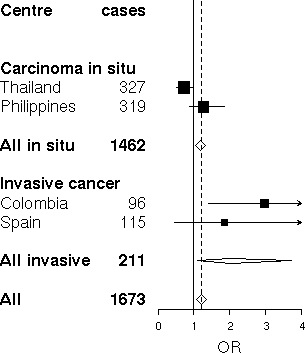or plots arranged in a tree (this can be seen as a generalization of
lattice plots, that present several facets of a dataset arranged on a
grid).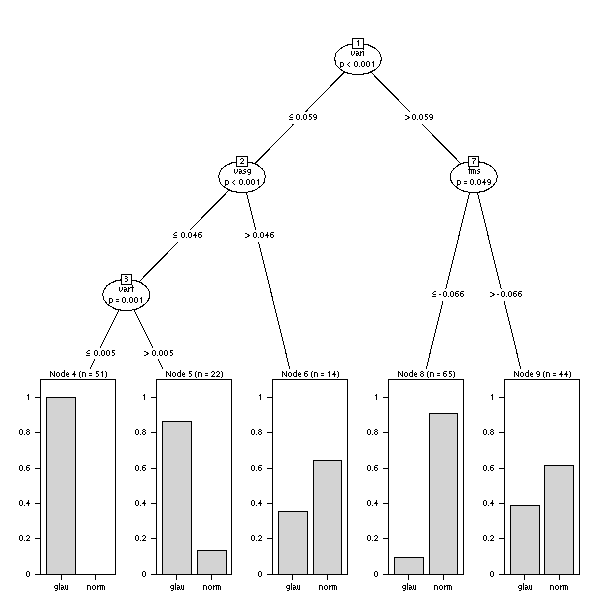One whole chapter is devoted to the creation, from scratch, of an
oceanographic plot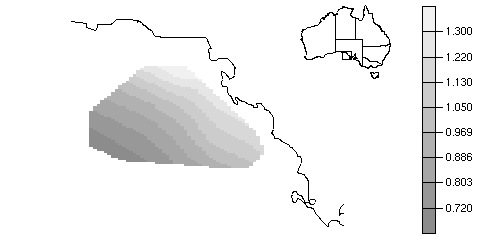whose elements are then reused for a completely different plot.See more ...